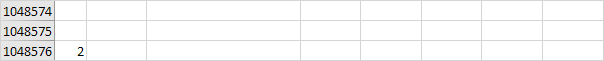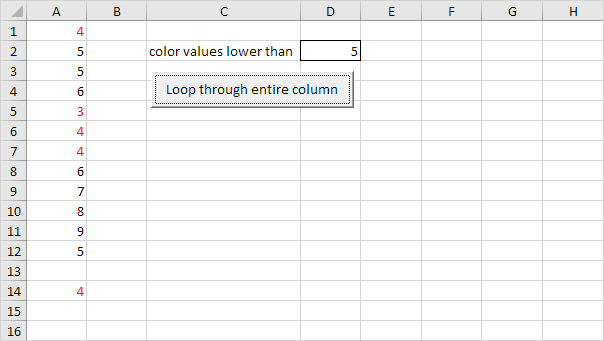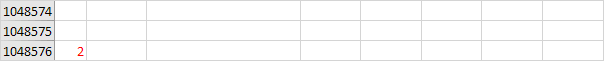# Loop through Entire Column in Excel VBA

Below we will look at a program in Excel VBA that loops through the entire first column and colors all values that are lower than a certain value.Place a command button on your worksheet and add the following code lines:

1. First, declare a variable called i of type Long. We use a variable of type Long here because Long variables have larger capacity than Integer variables.

Dim i As Long

2. Next, add the code line which changes the font color of all the cells in column A to black.

Columns(1).Font.Color = vbBlack

3. Add the loop.

For i = 1 To Rows.Count

Next i

Note: worksheets can have up to 1,048,576 rows in Excel 2007 or later. No matter what version you are using, the code line above loops through all rows.

4. Next, we color all values that are lower than the value entered into cell D2. Empty cells are ignored. Add the following code lines to the loop.

If Cells(i, 1).Value < Range("D2").Value And Not IsEmpty(Cells(i, 1).Value) Then
Cells(i, 1).Font.Color = vbRed
End If

Result when you click the command button on the sheet (this may take a while):Go to Next Chapter: Macro Errors